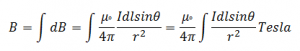# Biot-Savart Law

The Biot-Savart Law relates magnetic fields to the currents which are their sources. In a similar manner, Coulomb’s law relates electric fields to the point charges which are their sources. Finding the magnetic field resulting from a current distribution involves the vector product, and is inherently a calculus problem when the distance from the current to the field point is continuously changing.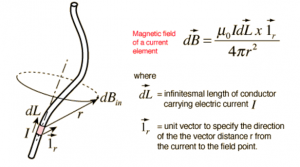Consider a conductor through which a current I flows and let small elemental length dl at a source point. We want to calculate magnetic field at a point which is distance r from source point. θ be the angle between r and dl. Then from biot-savart law the magnetic field due to current carrying conductor is
directly proportional to the magnitude of current,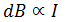directly proportional to the length of element dl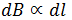directly proportional to the sine of angle between r and dl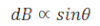inversely proportional to the square of the distance between source point and field point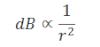Hence total magnetic field due to current carrying conductor is